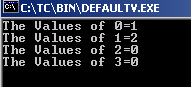Ads

Tutorials

# C Array default values

The example below contains an array arr of integer type. The maximum size of the array is 4.

The example below contains an array arr of integer type. The maximum size of the array is 4.

# C Array default values

In this section, you will learn how to set the default values in Array.

The example below contains an array arr of integer type. The maximum size of the array is 4. We have allocated only two values in the array like this:

int arr = {1,2};
Therefore we need to set the last two values of an array to a default value. We can do this with a for loop that sets the last values in the array to the default value. When the code is run, the pre-defined values with the indexes of the array is displayed and two zeroes as default values are set into the array with last blank or null indexes.

clrscr()- This method is defined in the header file <conio.h> which clears the console screen.

getch()- This method reads each character. Without this the output on the console can not be viewed.

Here is the code:

 ``` #include  #include  void main() {   clrscr();   int arr = { 1, 2 };   for (int i=0; i<4; ++i)   printf("The Values of %d=%d\n", i, arr[i]);   getch(); }```

Output will be displayed as:Download Source Code:

Ads

Ads